Question

Pspice/LTspice

8.83 To further increase the output resistance of a cascode current source or current mirror, another level of cascoding can be employed as shown is Fig. P8.83. Find the output resistance of this double-cascode current mirror.Verified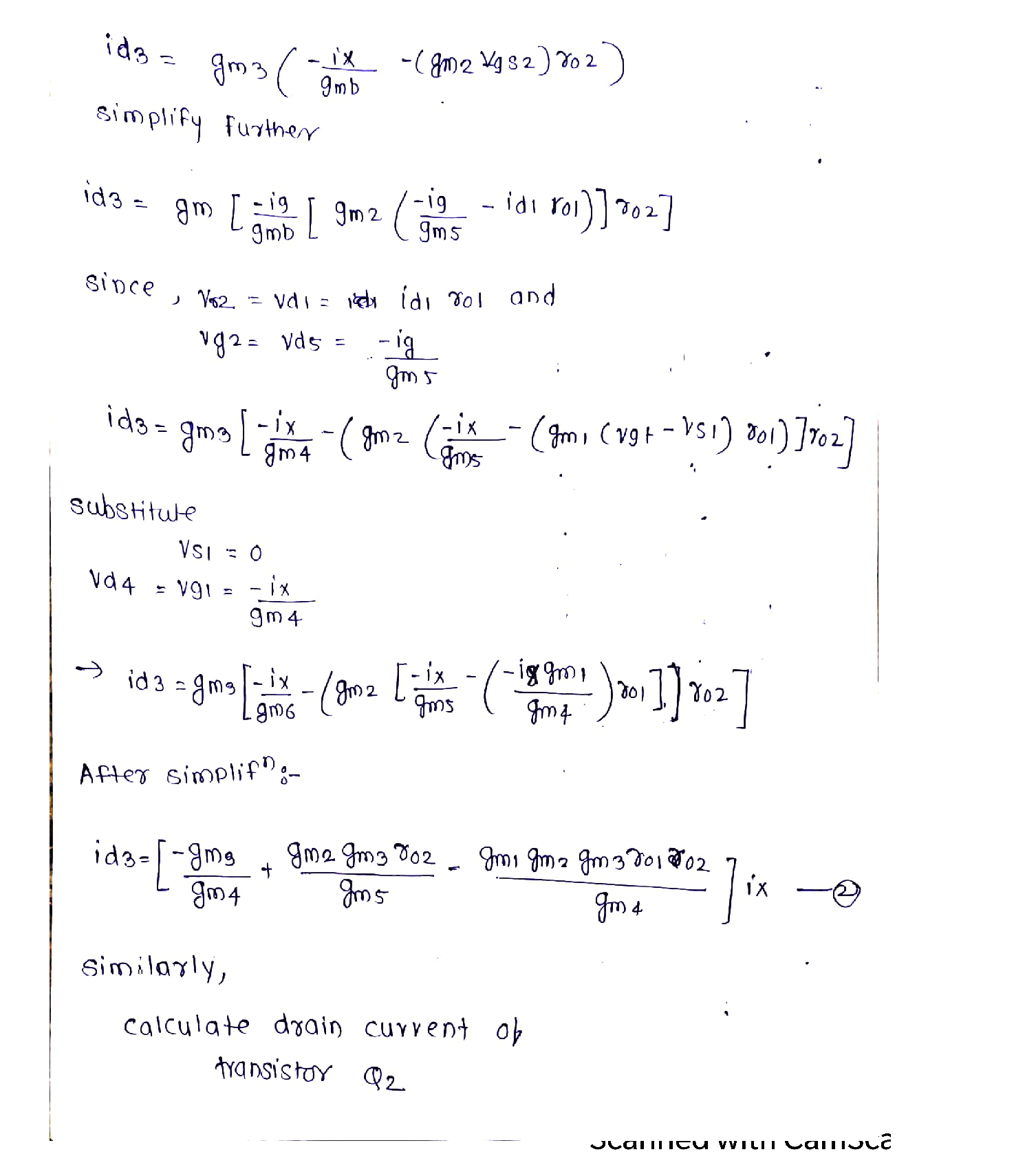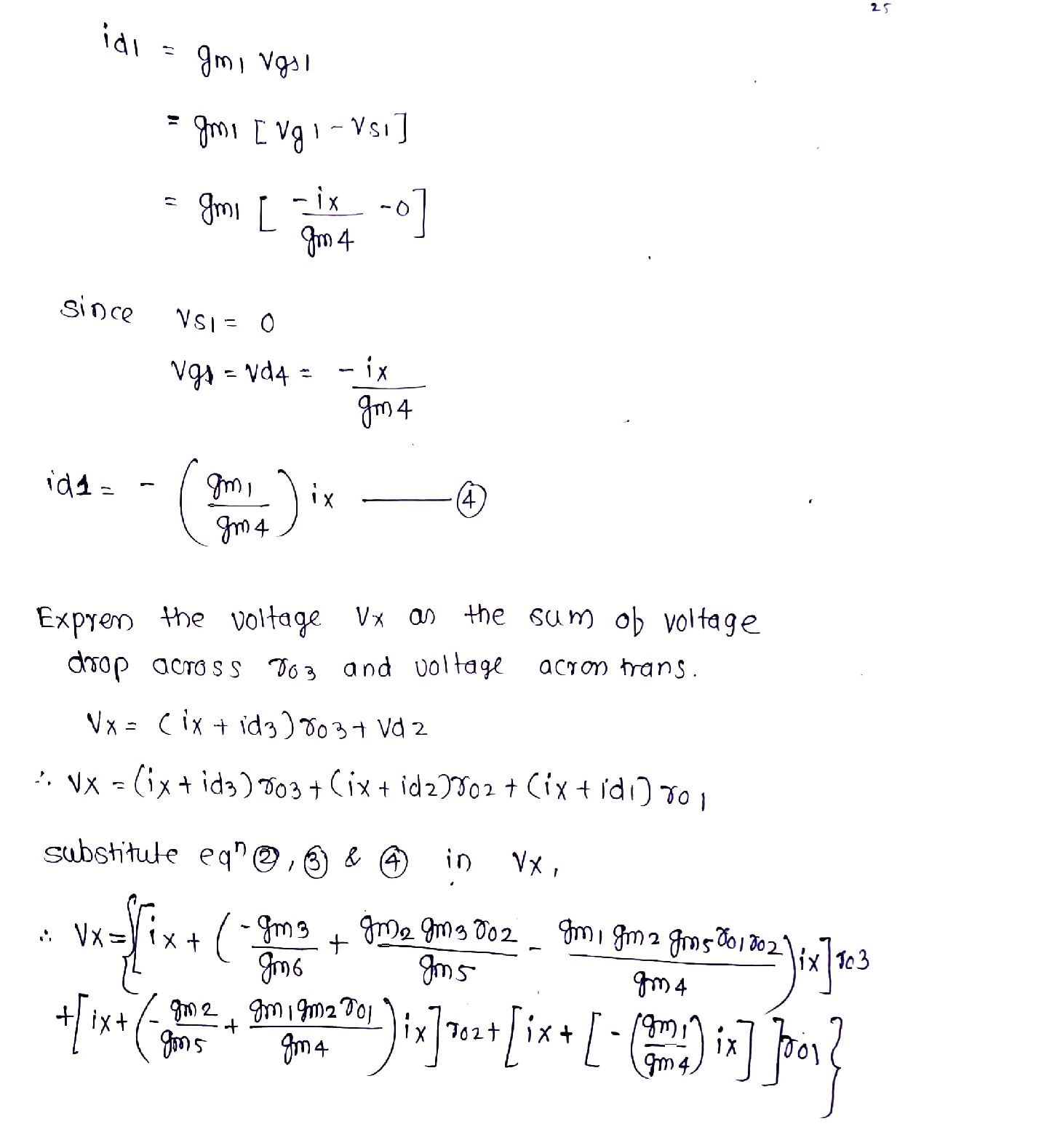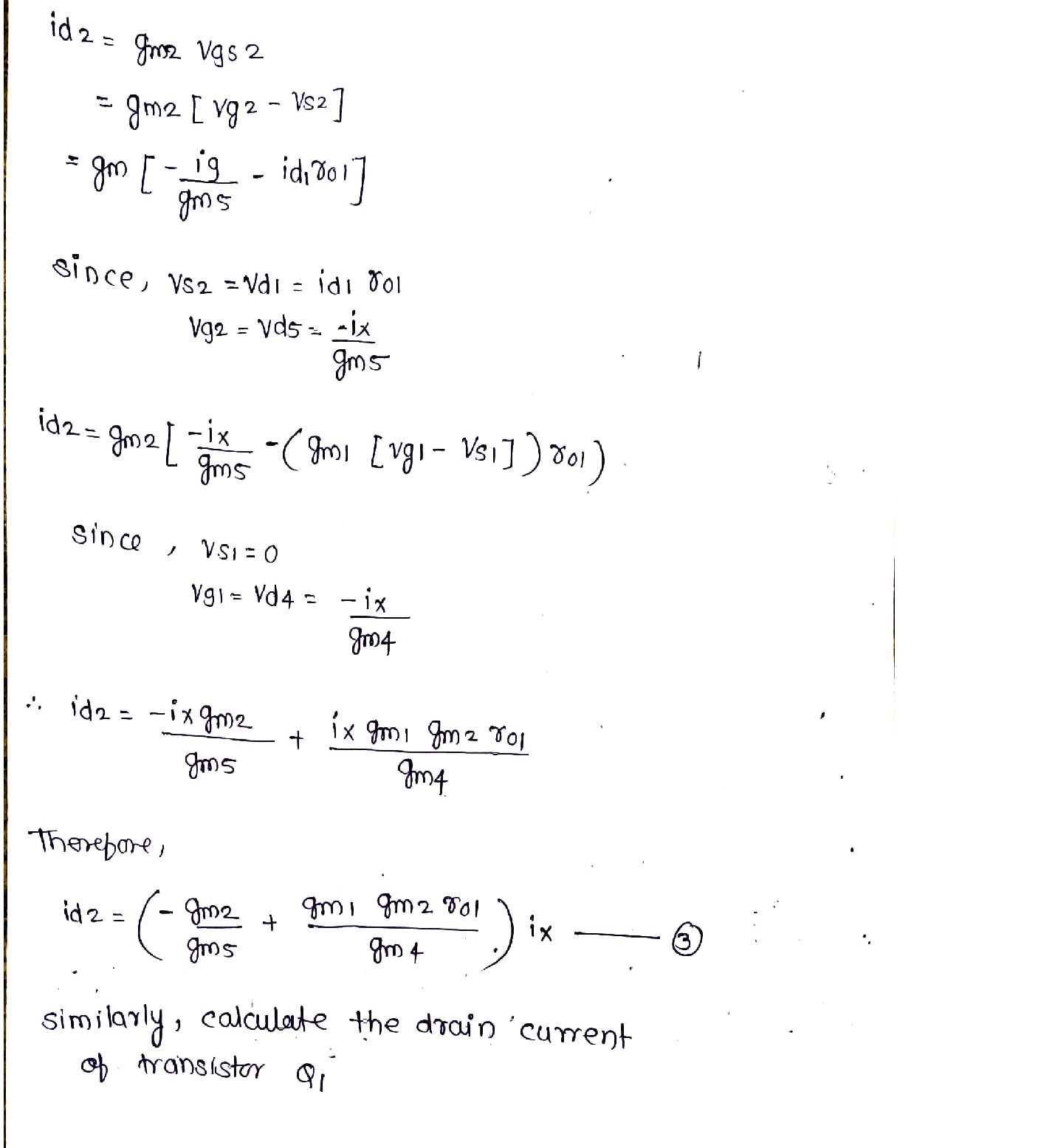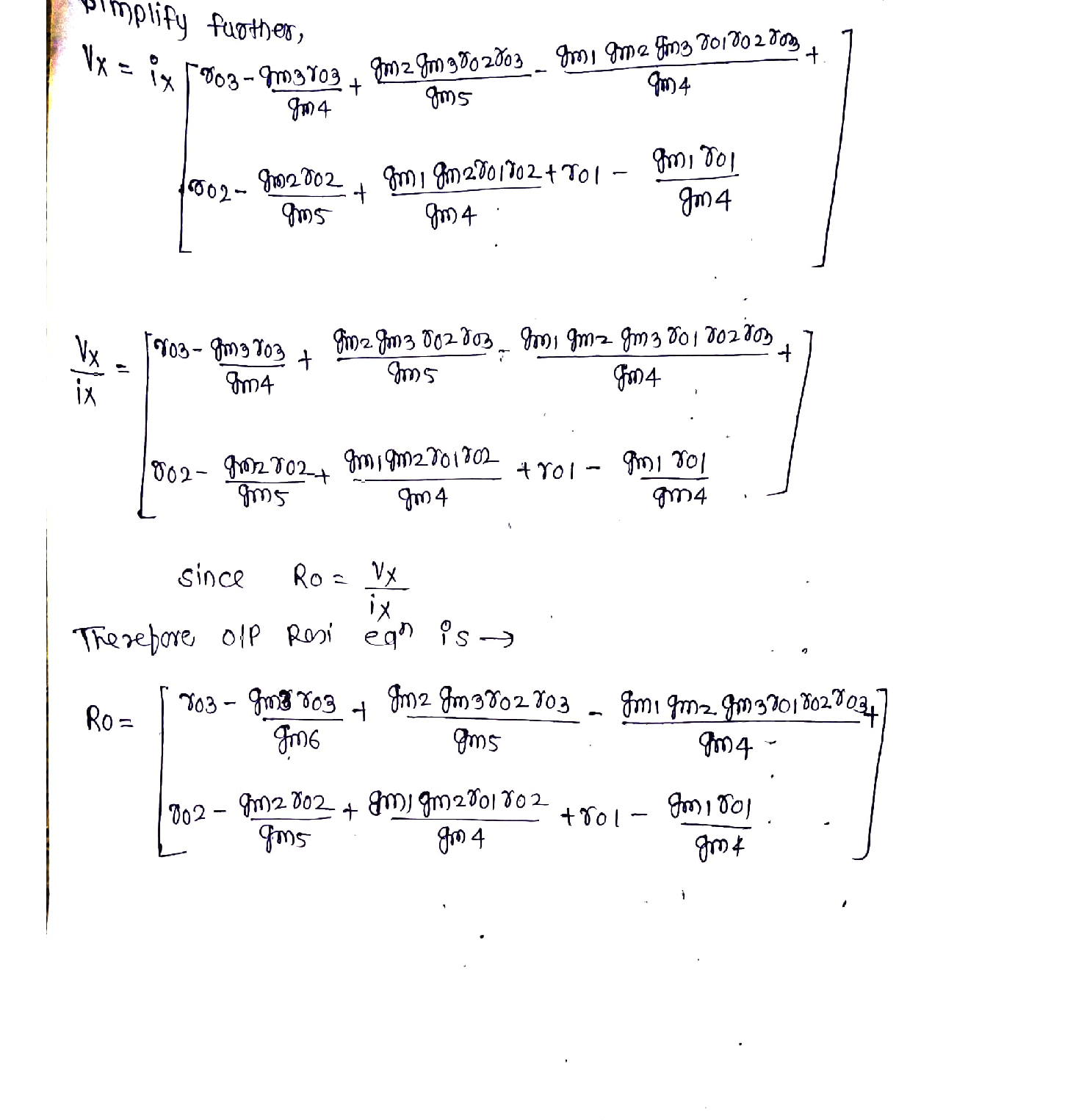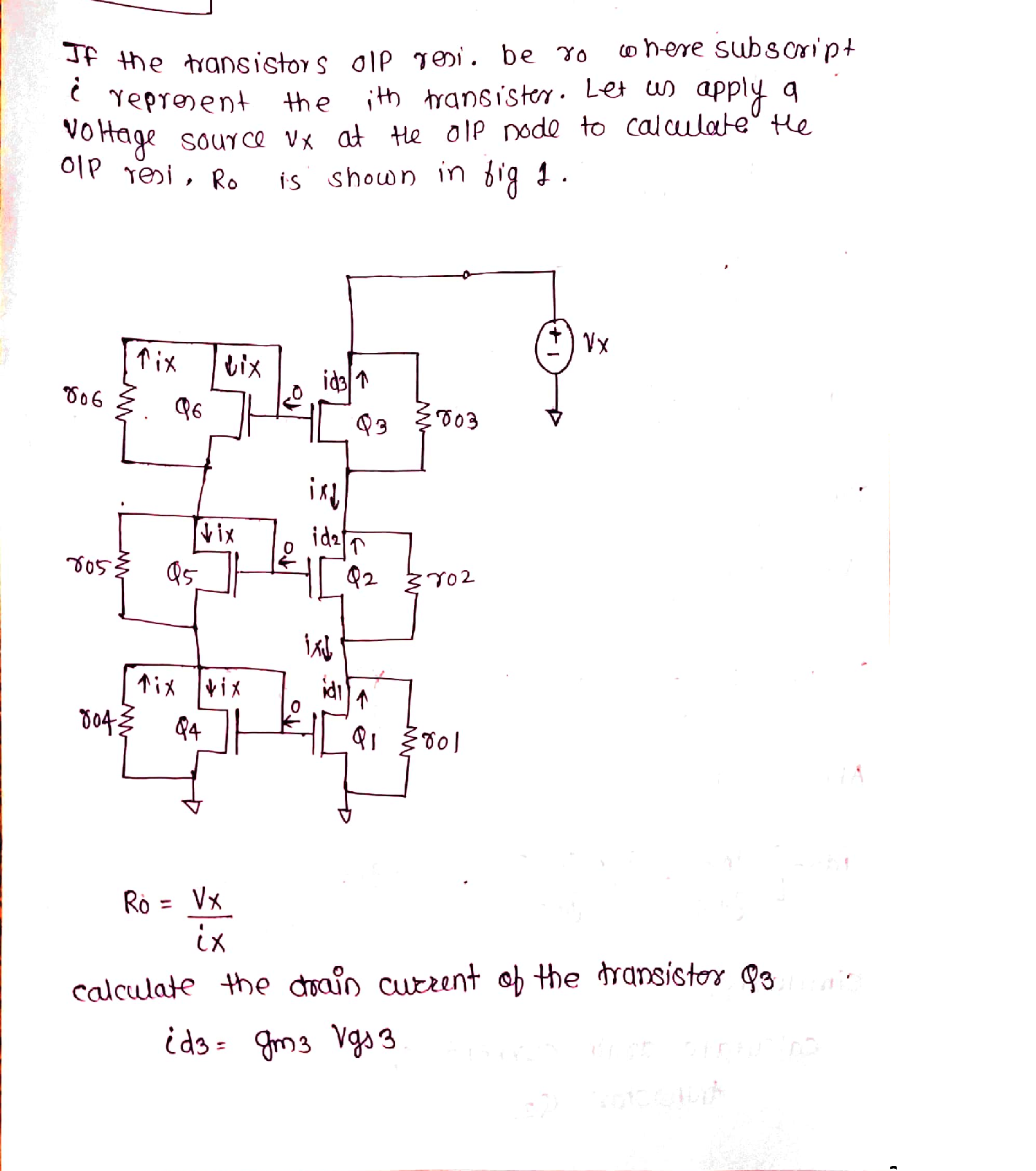### Question 37907Pspice/LTspice

8.91 Using the idea employed in the modified Wilson MOSmirror in Fig. 8.38(c), modify the Wilson BJT mirror of Fig.8.37(a) to eliminate the systematic error resulting from thedifference in VCE of Q, and Q.

### Question 37906Pspice/LTspice

8.63 The cascode amplifier in Fig. 8.28(a) is fabricated using two identical transistors having V, =3 V and operated at Voy = 0.15 V. What is the realized open-circuit voltage gain?

### Question 37904Pspice/LTspice

8.83 To further increase the output resistance of a cascode current source or current mirror, another level of cascoding can be employed as shown is Fig. P8.83. Find the output resistance of this double-cascode current mirror.

### Question 37903Pspice/LTspice

a) For the CG amplifier in Fig. 8.18(a), show that thecurrent gain i,/i is given by
\frac{i_{o}}{i_{\text {sig }}}=\frac{R_{\text {s }}}{R_{\text {s }}+R_{\text {in }}}
O For the case g, =2 mA/V, r, = 20 k2, and R, = 20 kN,find the current gain obtained with R,%3D20 kN.%3D
(c) If R, is doubled (that is, increased by 100%), find the corresponding percentage change in current gain.
If R, is increased from 20 kohms to 200 kohms (that is,increased by 900%), what is the corresponding change in current gain?
e) Using the results of (c) and (d), comment on the performance of the CG circuit as a current buffer.

### Question 37901Pspice/LTspice

8.73 The purpose of this problem is to investigate the signal currents and voltages at various points throughout a cascode amplifier circuit. Knowledge of this signal distribution is very useful in designing the circuit so as to allow for the required signal swings. Figure P8.73 shows a CMOS cascode amplifier with all de voltages replaced with signal grounds.
As well, we have explicitly shown the resistance r, of each-of the four transistors. For simplicity, we are assuming that-the four transistors have the same g and r,. The amplifier is fed with a signal v,.
-) Determine R,, R,, and R,. Assume gr, »I.
Determine i,, i, i,, i̟, iş, i̟, and i,, all in terms of v,.
If v, is a 5-mV peak sine wave and gmr0, = 20, sketch and clearly label the waveform of v1 v2, and v3.

### Question 37899Pspice/LTspice

8.68 The cascode amplifier of Fig. 8.31 is operated at acurrent of 0.2 mA with all devices operating at Vov =0.25 V. All devices have V A = 4 V. Find gm, the output resistance of the amplifier, R, the output resistance of recurrent source, the overall output resistance, R, and the voltage gain, A,.

### Question 37898Pspice/LTspice

(a) Neglecting the effect of V,. find the de drain current ofQ, and the required value of Vas:A
(b) Find the values of g and r, for all transistors.
) Find the value of R.in
(d) Find the value of R.out
out"Calculate the voltage gains v/v, and v,/vsig
How large can vsig be (peak-to-peak) while maintaining saturation-mode operation for Q1, and Q2?

### Question 37892Pspice/LTspice

8.34 For an NMOS transistor with L = 0.5 µm fabricated in the 0.13-um process specified in Table K.1 in Appendix K, find g r, and A, if the device is operated with Vov =0.15 V and 1,= 50 µA. Also, find the required device width W.

### Question 37891Pspice/LTspice

8.33 An NMOS transistor operated with an overdrive volt-age of 0.25 V is required to have a g equal to that of an npn transistor operated at I = 50 µA. What must I, be? What value of g, is realized?

### Question 37890Pspice/LTspice

8.30 An NMOS transistor fabricated in a certain process is found to have an intrinsic gain of 40 V/V when operated a tan I, of 100 µA. Find the intrinsic gain for 1p, = 25 µA and Ip, = 400 µ A. For each of these currents, find the factor by which g changes from its value at Ip = 100 µA.

### Submit query

Getting answers to your urgent problems is simple. Submit your query in the given box and get answers Instantly.

### Submit a new QuerySuccess

Assignment is successfully created